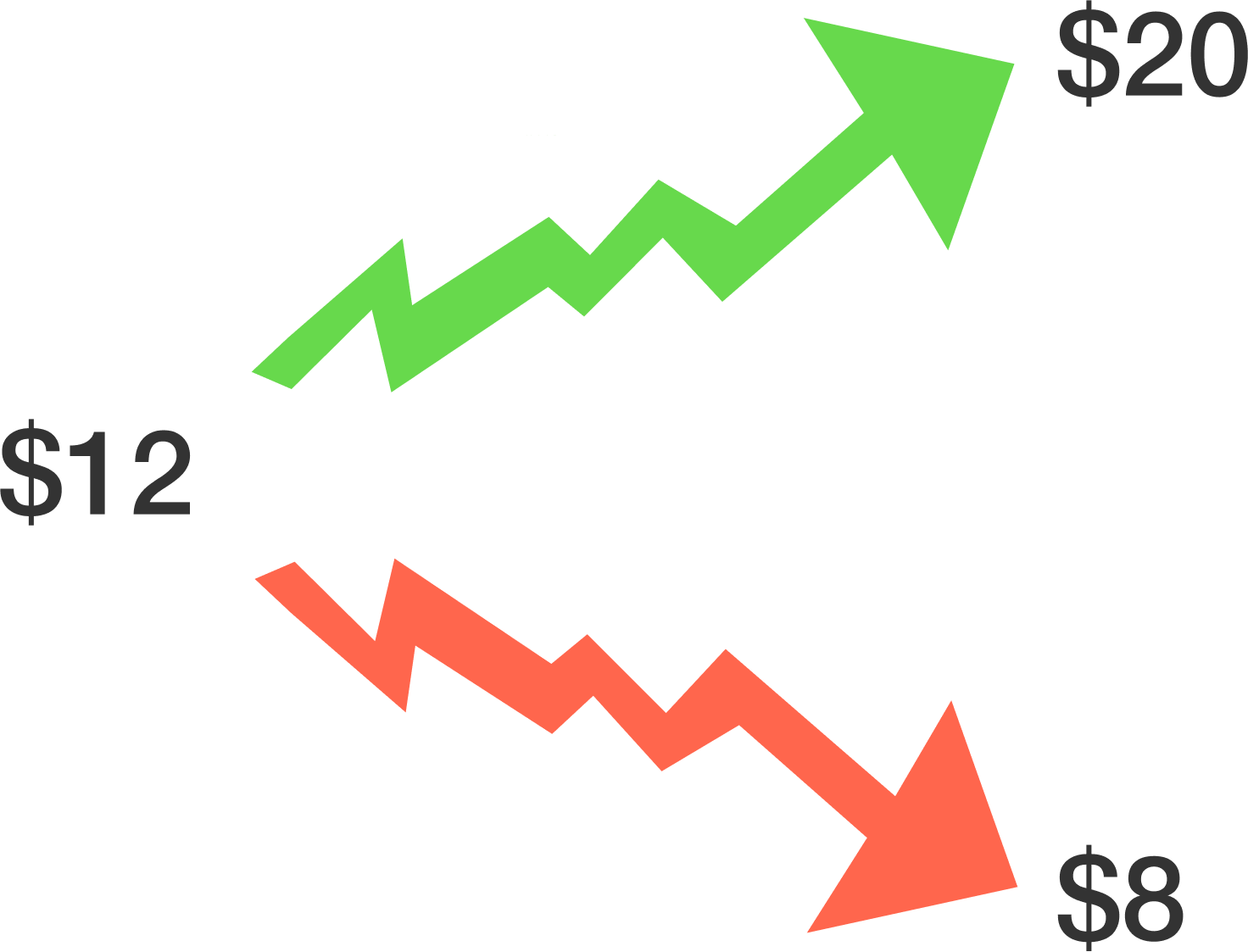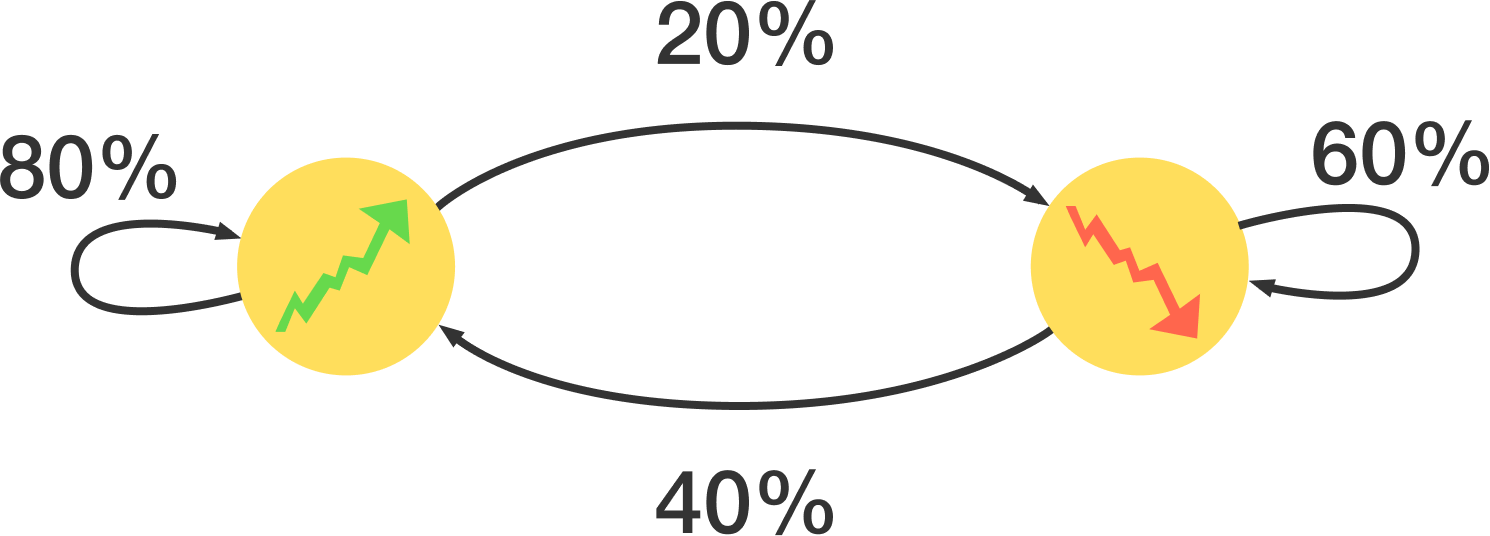### Math for Quantitative Finance

Probability and statistics are the foundation of most quantitative financial models. They allow us to interpret large amounts of data and model future outcomes in a way that humans could not do on their own.

# Probability

Financial firms often need to determine how various assets relate to each other, creating potentially profitable opportunities to trade both simultaneously. Which of the following pairs of stocks would you expect to have the greatest correlation?

# Probability

Models of stocks (or other assets) often include a consideration of how erratically the price moves. Using your intuition, which of these stocks had the highest average daily percent change in 2015?

# ProbabilityA biotechnology stock is currently trading at $12, and the company is releasing the results of a drug test tomorrow. An analyst tells you that if the test was successful, the stock should rise to$20; otherwise, it will fall to $8. If the current stock price is equal to its future expected value, what is the probability that the drug test will be announced as successful as implied by the analyst? # ProbabilityOver time, you have found that the price of a certain asset seems to follow a Markov model in which it will increase or decrease minute-to-minute according to the model above. For example, if its price increases one minute, it is 80% likely to increase again in the next minute. In the long run, in what portion of all minutes does the stock price increase? Hint: If it increases with probability $p,$ it decreases with probability $1-p.$ What value of $p$ would provide a steady state in this chain? # Probability Certain types of traders attempt to repeatedly buy and sell the same asset for a profit over a short time period, such as high-frequency “market makers”. For example, if you can repeatedly sell a stock for$8.50 and buy it for $8.49, you will make$0.01 each time.

If this transaction succeeds with probability 99%, about how many times can this transaction be executed before the probability of at least one failure exceeds 50%?×